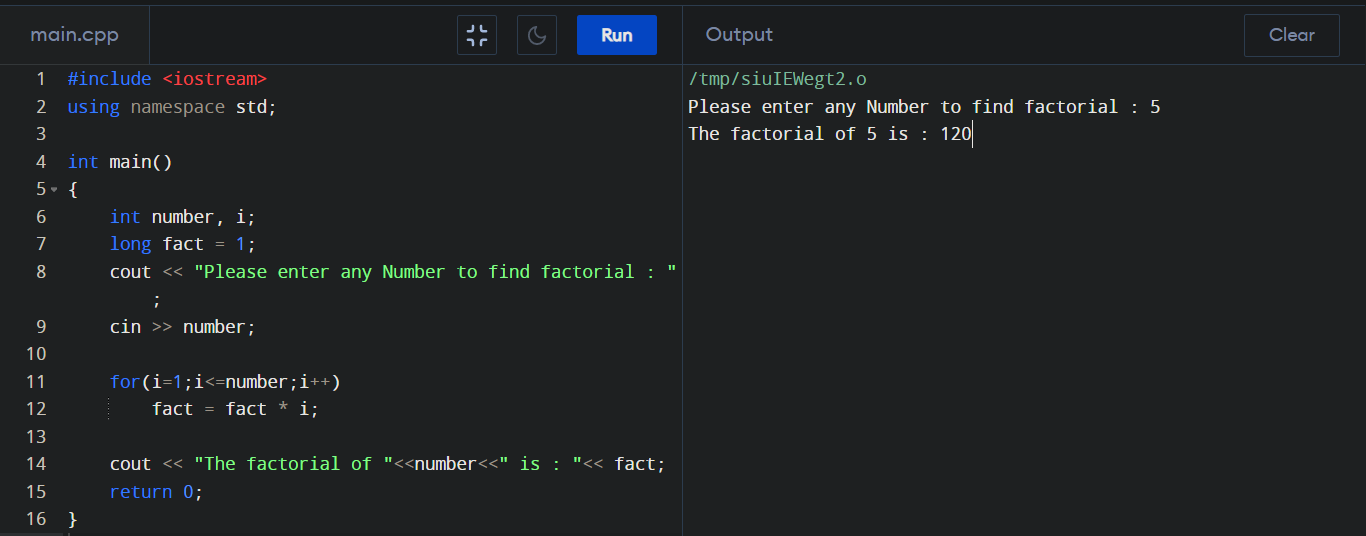C++ Program to find Factorial of a Number

Factorial of n is the product of all positive descending integers. Factorial of n is denoted by n!
For example, n! = n * (n-1) * (n -2) * …….* 1

Factorial in C++ : #include <iostream> using namespace std; int main() { int number, i; long fact = 1; cout << "Please enter any Number to find factorial : "; cin >> number; for(i=1;i<=number;i++) fact = fact * i; cout << "The factorial of "<< number <<" is : "<< fact; return 0; } Output :